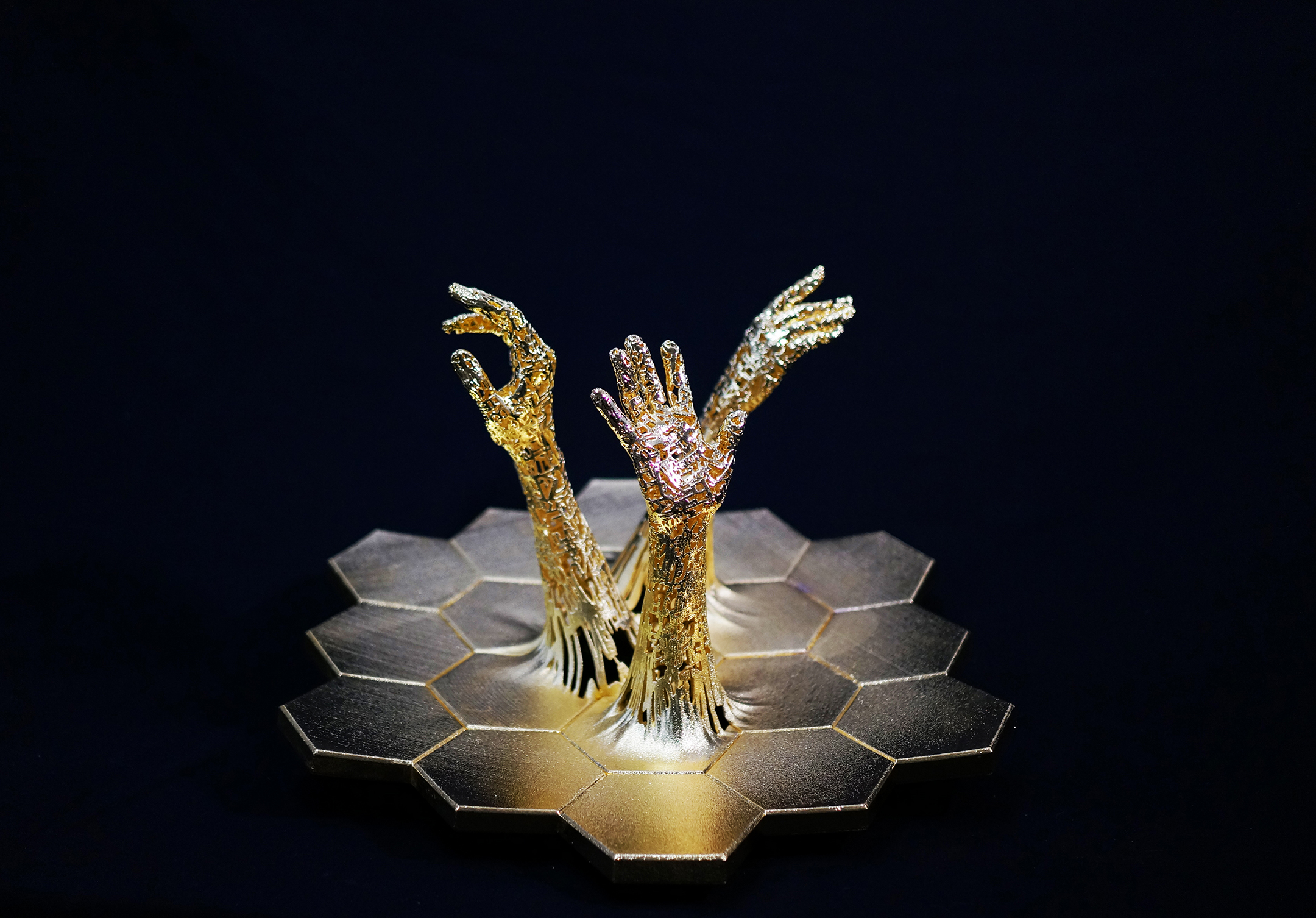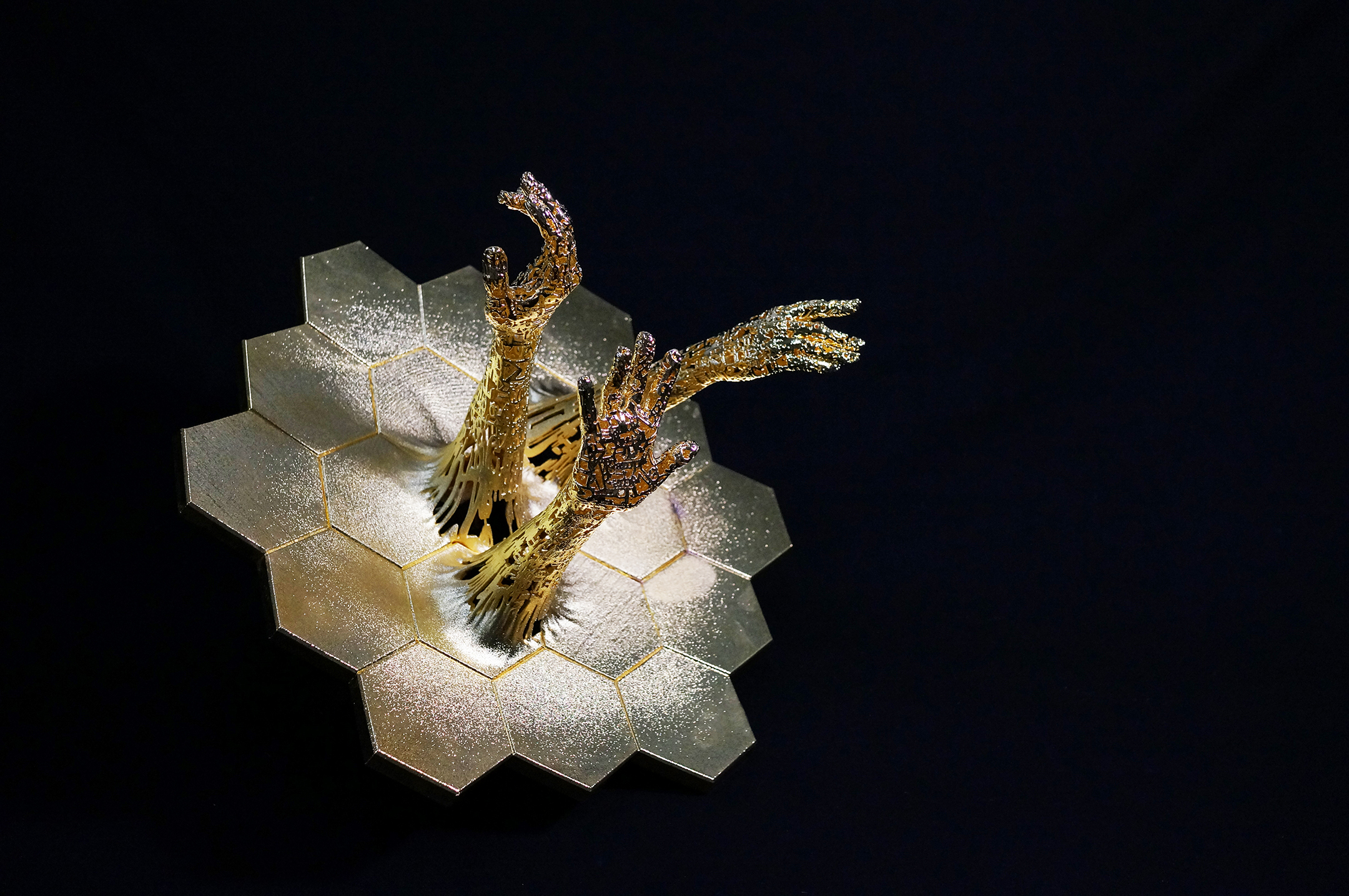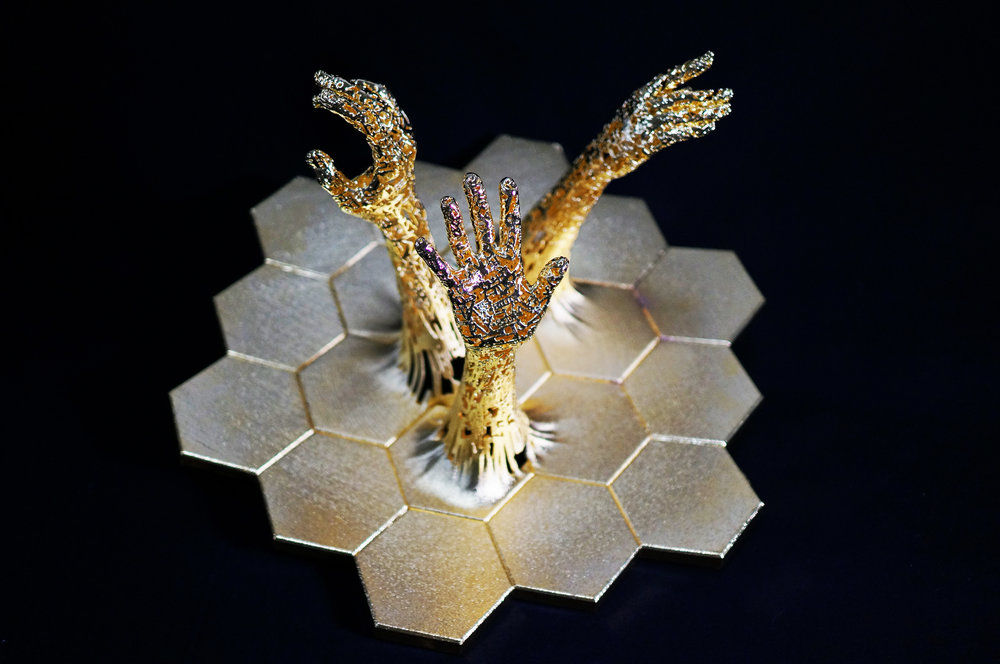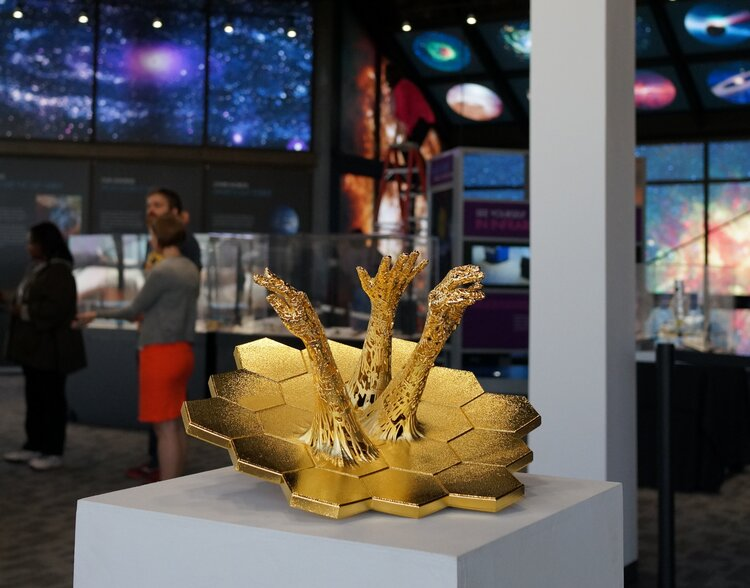No items found.

### Exploration JWST

2017
17 in x 17 in x 13 in (43 cm x 42 cm x 33cm)
NASA Laser gold plated 3D printed nylon

in collaboration with NASA Goddard Space Flight Center

Exploration. The sole purpose of the James Webb Space Telescope mission. To look back in time and see how our universe was formed. To raise questions we never thought to ask. To reach into the unknown.

“Astronomy by its very nature drives us toward the unknown…there’s something uniquely human about wanting to find out about our surroundings, to explore our world, to discover new things.  That’s what astronomy is all about." - Amber Straughn

Exploration was created using 3D scans of John Cromwell Mather, astrophysicist, cosmologist and Nobel Prize in Physics laureate and Amber Straughn Astrophysicist; Deputy Project Scientist for JWST Science Communications. The arms are reaching out of the primary mirror of the JWST as though they are reaching through a portal to the ends of the universe. As they push through they are enveloped in information, formulas that have helped unlock the secrets of the universe.

John describes the Friedmann–Lemaître–Robertson–Walker metric which is used to wrap the arms of the piece. This metric is an exact solution of Einstein's field equations of general relativity.

“The equations wrapped around the hands in this sculpture are the “FLRW" cosmological solution to Einstein’s field equations of general relativity, paired with the formula that describes a parabolic mirror.  One might say we build one (the telescope primary mirror) to test the other (Einstein’s equations).

Einstein gave us the first equations that could be used to describe the gravitation of an infinite universe, which seems to be where we live.  His equations of general relativity for this describe the universe in terms of curvature and distance in space time, as well as the effect of matter and radiation on gravity.

The “metric tensor,” one mathematical variable in these equations, has this meaning:  a particle of matter will follow a path that makes the total “distance” between the two endpoints in space-time the smallest. Curiously enough we have a very similar principle for light.

For light, the “distance” between two endpoints in space-time is zero. (Relativity is a little surprising.) However in ordinary three dimensions of space, light follows the path that makes the total distance smallest. We can actually design our telescope so that light from a distant star goes through our telescope with the same total distance from star to focal point, regardless of where the light hits the primary mirror. We do this by making our primary mirror curve inward, in a shape called a parabola.

There are cosmological solutions to Einstein’s field equations that can be used to mathematically describe the universe. The FLRW one (so named because it was derived by scientists named Friedmann, Lemaître, Robertson, and Walker) gives us a model of a universe with a Big Bang, which approximates the universe we can observe with our telescopes.  The equations of this cosmological solution use variables like: change in size of the universe over time; density; and pressure. There is also a number in these equations related to the “size” of the universe - though if the universe is infinite, that can’t be the whole story.   In the end, what we are doing is pairing observation with theory to try to create a better description of the universe we live in.”

The third arm is my own; the arm of an artist. The abstract idea of studying what you don't know is hard to grasp. This is a disconnect that art can help fill in. Art asks people every day to think about abstract ideas and opens a doorway for creative thinking. My hope is to apply this open mindedness to science and in this way be better equipped to take in the universe in all its vastness and mystery.

Special thanks to NASA Goddard, Maggie Masetti, John Cromwell Mather, and Amber Straughn.

### Exploration JWST

2017
17 in x 17 in x 13 in (43 cm x 42 cm x 33cm)
NASA Laser gold plated 3D printed nylon

in collaboration with NASA Goddard Space Flight Center

Exploration. The sole purpose of the James Webb Space Telescope mission. To look back in time and see how our universe was formed. To raise questions we never thought to ask. To reach into the unknown.

“Astronomy by its very nature drives us toward the unknown…there’s something uniquely human about wanting to find out about our surroundings, to explore our world, to discover new things.  That’s what astronomy is all about." - Amber Straughn

Exploration was created using 3D scans of John Cromwell Mather, astrophysicist, cosmologist and Nobel Prize in Physics laureate and Amber Straughn Astrophysicist; Deputy Project Scientist for JWST Science Communications. The arms are reaching out of the primary mirror of the JWST as though they are reaching through a portal to the ends of the universe. As they push through they are enveloped in information, formulas that have helped unlock the secrets of the universe.

John describes the Friedmann–Lemaître–Robertson–Walker metric which is used to wrap the arms of the piece. This metric is an exact solution of Einstein's field equations of general relativity.

“The equations wrapped around the hands in this sculpture are the “FLRW" cosmological solution to Einstein’s field equations of general relativity, paired with the formula that describes a parabolic mirror.  One might say we build one (the telescope primary mirror) to test the other (Einstein’s equations).

Einstein gave us the first equations that could be used to describe the gravitation of an infinite universe, which seems to be where we live.  His equations of general relativity for this describe the universe in terms of curvature and distance in space time, as well as the effect of matter and radiation on gravity.

The “metric tensor,” one mathematical variable in these equations, has this meaning:  a particle of matter will follow a path that makes the total “distance” between the two endpoints in space-time the smallest. Curiously enough we have a very similar principle for light.

For light, the “distance” between two endpoints in space-time is zero. (Relativity is a little surprising.) However in ordinary three dimensions of space, light follows the path that makes the total distance smallest. We can actually design our telescope so that light from a distant star goes through our telescope with the same total distance from star to focal point, regardless of where the light hits the primary mirror. We do this by making our primary mirror curve inward, in a shape called a parabola.

There are cosmological solutions to Einstein’s field equations that can be used to mathematically describe the universe. The FLRW one (so named because it was derived by scientists named Friedmann, Lemaître, Robertson, and Walker) gives us a model of a universe with a Big Bang, which approximates the universe we can observe with our telescopes.  The equations of this cosmological solution use variables like: change in size of the universe over time; density; and pressure. There is also a number in these equations related to the “size” of the universe - though if the universe is infinite, that can’t be the whole story.   In the end, what we are doing is pairing observation with theory to try to create a better description of the universe we live in.”

The third arm is my own; the arm of an artist. The abstract idea of studying what you don't know is hard to grasp. This is a disconnect that art can help fill in. Art asks people every day to think about abstract ideas and opens a doorway for creative thinking. My hope is to apply this open mindedness to science and in this way be better equipped to take in the universe in all its vastness and mystery.

Special thanks to NASA Goddard, Maggie Masetti, John Cromwell Mather, and Amber Straughn.No items found.